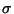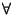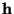Next:  Examples Up:  Methods, algorithms and a Previous:  Methods, algorithms and a   Contents

## The method

The question is : Given a set of incomplete and noisy data (say, Fo,with its(F) and), which map (of a large number of maps consistent with the observed data) is the one that will minimise the probability of misinterpreting it ? Stating the same problem in a different way, we could ask (i) which map (of the set of admissible maps) will only show features for which there is evidence in the data, or, (ii) which map makes the least assumptions about the data (especially the missing data, but also the distribution of errors in the observed).

Clearly, the Fo,exp(i) synthesis is not the map we want : Not only we assume that all missing data have F = 0 (a rather improbable event), but also that F= Fo,,. Gull, S.F. & Daniell, G.J.4, suggested that the map we need is the one for which the configurational entropy -mjlogmj, where mj is the density at the grid point j of the map, reaches a maximum. It is easy to see that -mjlogmj reaches a maximum when mj = e-1,j, that is, when the map has a uniform density, and thus, contain no information. Maximising -mjlogmj subject to the constraint that the map is consistent with the observed data, gives the MAXENT map.

The consistency with the observed data is described in terms of the difference between the observed data and those calculated from a trial map, weighted by the standard deviation of the measurement. If Fc,is the calculated value of the datum h, Fo,its observed value and(F) the standard deviation of the observation, then the statisticpossesses adistribution with an expected value equal to the number of data points. Maximising -mjlogmj subject to the constraint| Fc,- Fo,/(F)2 = n, where n is the number of data points, gives the basic iteration formula :

m= exp{ - 1 +exp(2i)}

Given Fo,,(F) and an positive multiplier, this equation can determine the densities mon a map. The program GraphEnt applies this formula iteratively (starting from a uniform map) until convergence (as judged by the value of) is achieved. Although this algorithm is neither the most efficient nor the most stable, it is relatively easy to code and it leads (at least in the case of Patterson functions), to the same results as other, more complex algorithms5.

#### Footnotes

... G.J.4
Gull, S.F. & Daniell, G.J., (1978), Nature, 272, 686-690.
... algorithms5
Skilling, J. & Bryan, R.K., (1984), Mon. Not. R. astr. Soc., 211, 111-124.Next:  Examples Up:  Methods, algorithms and a Previous:  Methods, algorithms and a   Contents
NMG, Nov 2002